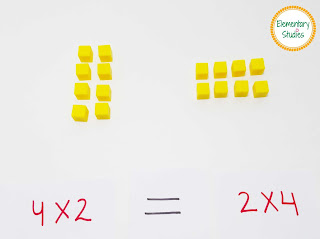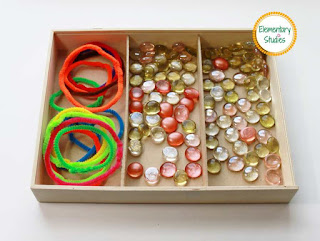## Elementary Studies

### Division Facts

Division is one of the four basic operations of arithmetic, the other being addition, subtraction, and multiplication. Once kids are fluent in multiplication facts, teaching division becomes easy. My today’s post is on introducing the concept of division to the young learners.

#### Introducing Division

Division is a sharing operation, where objects are shared equally into a number of groups.

Hands on Activity: Give 9 pennies or counters and 3 bowls to each child and tell them to put equal pennies/counters in each bowl.

If the result is as shown in picture, tell them - This is what the division is.

#### Multiplication and Division relationship (Multiplication and Division Fact Family)

Multiplication and Division has inverse relationship just like addition and subtraction.

Example:
Multiplication Equation:  4 x 5 = 20
The two division equations will be:
20 ÷ 5 = 4
20 ÷ 4 = 5

Similarly, we can derive multiplication equations from division equation.
35 ÷ 5 = 7

The two multiplication equations will be:
7 x 5 = 35
5 x 7 = 35

Understanding the multiplication and division relationship is very important in solving the division problems.

#### Division Vocabulary

Dividend: A number to be divided by another number.
Divisor: A number by which another number is divided.
Quotient: The result of division.

This division vocabulary poster is also included in my division packet.

You can use this division booklet to teach division facts up to 12 without a remainder. Worksheets covering the above concepts are included in this packet.

This division packet can be purchased from HERE

Happy Teaching!

Shimps

### Properties of Multiplication

In continuation of my posts on the Multiplication topic, today I will be sharing my work on Properties of Multiplication. As per common core standards a 3rd grader is expected to know about these multiplication properties which includes Associative property, Commutative property, Distributive property, Identity property, and Zero property.

This week when I was teaching this topic, I had my kiddo first use the blocks to visualize and see these multiplication properties in action. I am a big fan of these place value blocks and it always comes handy. I bought mine from Lakeshore, but these are now available at Amazon for less 😊. You can get these at Amazon: Place Value Blocks

Later, I gave her the worksheets (HERE) to reinforce her understanding of Properties of Multiplication.

Associative Property of multiplication states that you can change the grouping of the factors the product will be the same.
Example: (5 x 2) x 3 = 30
5 x (2 x 3) = 30

Commutative Property of multiplication states that you can multiply the factors in any order, the product stays the same.
Example: 4 x 2 = 2 x 4Commutative Property of Multiplication

### Multiplication Activities

Hands-on activities are very important in teaching math concepts. Research have shown that students presented with an opportunity to use manipulatives are more interested in mathematics. Today, I am sharing some fun hands-on ways to teach multiplication. Hope you can use and implement some of these ideas in your classroom.

### Multiplication as Equal GroupsMultiplication as Equal Group Activity Box

I bought these pipe cleaners from a Dollar Store. It is super easy to make the hoops with pipe cleaners. Just make a circle and twist at the ends. Ta-da your hoops are ready! For counters, I have used decorative stones which I bought from Michaels (during summer clearance 😊).  You may as well use Buttons, Pom-Poms, M&Ms, Paper Clips, Lego blocks etc.

### Multiplication Facts

Wow! What an amazing feeling that my blog is live. Happy  Happy Happy! Special thanks to my husband, you are amazing!

Now moving on to my next post, the question was what should I blog next? During this time of year in many classrooms, kids are learning Multiplication, so this became an obvious choice. Multiplication is a very important concept in Mathematics and just one post will not do justice to this topic, nor to my readers. 😊

Different strategies on multiplication are taught in the classroom; like equal group, repeated addition, Arrays, and Number line. Along with teaching these strategies, memorizing and automacy of multiplication facts is extremely important.

Multiplication is typically introduced in Grade 3, and covers a major part of the curriculum. In the first part of my multiplication post series, I am including Multiplication facts 0 to 12

I have structured my worksheets to build a foundation on this topic and help students on their journey of learning multiplication facts.